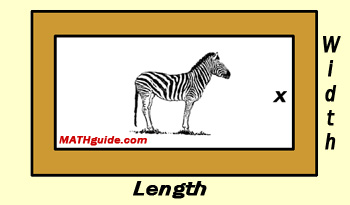Picture Frame Problem
View the Lesson | MATHguide homepage Updated December 18th, 2016

Status: Waiting for your answers.Kris is going to use a frame that is 1 inch thick for this job. This picture has a length that is 3 inches longer than it is wide. The area of the frame alone is given by this expression:

(x + 5)(x + 2) - (x + 3)x

There are four descriptions below. Use the pulldown menu to select the correct expression for each description:

 the length of the picture alone, in inches xx + 1x + 2x + 3x + 4x + 5x + 6x + 7x + 8 the length of the frame, in inches xx + 1x + 2x + 3x + 4x + 5x + 6x + 7x + 8x + 9x + 10 the area of the picture alone, in square inches (x)x(x + 1)x(x + 2)x(x + 3)x(x + 4)x(x + 5)x(x + 6)x(x + 7)x(x + 8)x the area of the picture and the frame together, in square inches (x + 4)(x + 1)(x + 5)(x + 2)(x + 6)(x + 3)